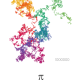# Different Update MethodsSciencificity

Hi James,

Here again I believe that the `symmetric_difference_update()` only works on 2 sets for a reason (hence the ^= operator too, should be used only with 2 sets)

If we take the 3 set example used in the videos, and introduce a 3 in the last set we notice the weird behaviour for more than 2 sets.

``````a = {1,2,3}
b = {3,4,5}
c = {1,3,5,6}

a ^= b ^ c
print(a)

{2, 3, 4, 6} # result

# 3 is in every set so should not be here in the result
# 3 gets eliminated in the a ^= b step (result = {1,2,4,5}),
# then reintroduced in the last ^c step ...
``````

Well, that’s my thinking anyway. Please let me know if I am thinking about it incorrectly?

Thanks for a really great course! It is just this concept which is confusing for me.James Uejio RP Team

@Sciencificity Thank you for the comment and this is definitely tricky. So what actually happened is Python evaluated the right hand side of the expression first (b ^ c) and then modified a.

You can think of it like this:

``````a = {1,2,3}
b = {3,4,5}
c = {1,3,5,6}

# step 1
print(b ^ c)
{1, 4, 6}

# step 2
print(a ^ {1, 4, 6})
{2, 3, 4, 6}

# step 3
Modify a to be equal to {2, 3, 4, 6}
``````Victoria

``````The explanation in the video is not correct. A counter-example, by adding another set.
a = {1, 2, 3}
b = {3, 4, 5}
c = {1, 5, 6}
d = {1} # additional set

a ^= b ^ c ^ d
# {1, 2, 4, 6}
``````

The `^` operations are chained and evaluated on the right first, before the final operation and assignment.

to join the conversation.

Lesson Completed!
Lesson Bookmarked
Request Failed :(